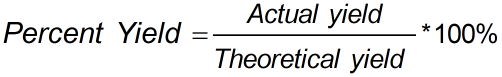Request a Tool

Percent Yield Calculator

To calculate the percent yield for the given amount of actual and theoretical yield

Percent Yield
0

FormulaDefination / Uses

Percent yield calculator is tool that finds the percent yield for the given amount of actual and theoretical yield.

Percent yield is the percent ratio of an actual yield to a theoretical yield. The percentage yield is frequently less than 100%. Chemistry's percent yield calculator is an online tool for calculating the percent yield of a chemical reaction. The percent yield calculator saves the user a lot of time when it comes to calculating results. The percent yield indicates how well you executed your reaction. Your percent yield would be 100 percent if you were really careful with your reaction, making sure that every molecule reacted and that nothing was lost as you poured the solution from one beaker to another (don't worry if you don't reach 100 percent, it's nearly impossible). Use the upper given formula for manual calculations. No sign-up, registration OR captcha is required to use this tool.